Previous: 2.2.2.2 Odd Moments Up: 2.2.2 Hierarchy of Moment Next: 2.2.3 Closure Problem

### 2.2.2.3 Non-Parabolicity Corrections

So far we have assumed parabolicity in our derivations. The non-parabolic case is discussed in broad detail in [GJK+04]. Here we restrict ourselves to a short summary.

First we have to decide on a set of solution variables and express the remaining moments via closure relations. A second issue is the choice of weight functions and indeed different sets of weight functions have been frequently used.

As solution variables we choose the unknowns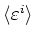and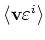, that is the moments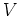. In the non-parabolic case we use the following set of weight functions: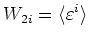(2.43)

and(2.44)

Note that the weight functions use the momentum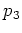in place of the velocity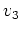. We then apply the weight functions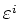and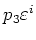to the Boltzmann equation.

The product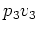, which appears in the calculation, has units of energy. In the derivation above, valid for the parabolic case, we used the fact that (under the isotropy condition) moments of the form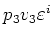can be reduced to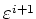. For non-parabolic bands this is no longer the case.

Due to the choice of solution variables the balance equations are independent of the band structure model and the same as in the parabolic case. For the flux equations a simple method to close the equations in the solution variables is to introduce correction factors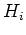, see [GJK+04]. This gives the following modified equations: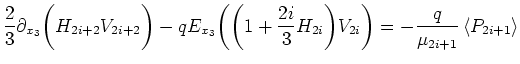(2.45)

where the integral from the scattering operator was modeled using mobilities, which will be discussed in Section 2.3.

The correction factors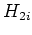can be tabulated as a function of temperature and doping extracted from bulk Monte Carlo simulations. We defineby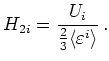(2.46)

This uses the quantity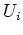which is given through the trace of the tensor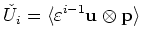(2.47)

by assuming cylindrical symmetry as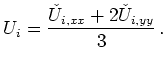(2.48)